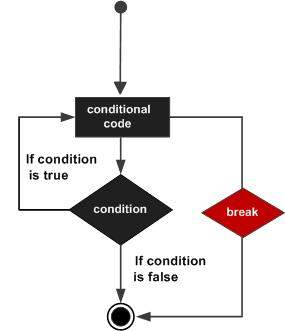# C# - Break Statement

The break statement in C# has following two usage −

• When the break statement is encountered inside a loop, the loop is immediately terminated and program control resumes at the next statement following the loop.

• It can be used to terminate a case in the switch statement.

If you are using nested loops (i.e., one loop inside another loop), the break statement will stop the execution of the innermost loop and start executing the next line of code after the block.

## Syntax

The syntax for a break statement in C# is as follows −

```break;
```

## Flow Diagram## Example

```using System;

namespace Loops {
class Program {
static void Main(string[] args) {
/* local variable definition */
int a = 10;

/* while loop execution */
while (a < 20) {
Console.WriteLine("value of a: {0}", a);
a++;

if (a > 15) {
/* terminate the loop using break statement */
break;
}
}
}
}
}
```

When the above code is compiled and executed, it produces the following result −

```value of a: 10
value of a: 11
value of a: 12
value of a: 13
value of a: 14
value of a: 15
```
csharp_loops.htm